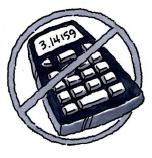Home > CC1MN > Chapter cc21 > Lesson cc21.2.1 > Problem1-62

1-62.

Add or subtract.  Remember to line up the decimal point.   Homework Help ✎1. $53.199−27.61$

Line the problem up vertically.

Add a zero and subtract.

Continue subtracting going from right to left.

Borrow 1 from the 3. Then subtract 6 from 11.

Borrow again.

$\left. \begin{array} \\\ \quad 53.199 \\ -27.61 \\ \hline \\ \end{array} \right.$

$\left. \begin{array} \\\ \quad 53.199 \\ -27.610 \\ \hline \qquad \ \ \ \ 9\end{array} \right.$

$\left. \begin{array} \\\ \quad 53.199 \\ \ -27.610 \\ \hline \qquad \quad \ 89\end{array} \right.$

$\left. \begin{array} \\\ \quad 5^2\cancel{3}.^1199 \\ \ -27.610 \\ \hline \qquad \ \ 589\end{array} \right.$

$\left. \begin{array} \\\ \quad ^4\cancel{5}^2\cancel{3}.^1199 \\ \qquad \ -27.610 \\ \hline \qquad \qquad \ \ 589\end{array} \right.$

2. $155.96+56.232$

Add from right to left, carrying when necessary. Now finish the problem.

$\left. \begin{array} \\ \ 155.96 \\ \ +56.232 \\ \hline \\ \end{array} \right.$

$\left. \begin{array} \ \small{1}\\ \ \ \ 155.960 \\ \ +56.232 \\ \hline \qquad \ .192\end{array} \right.$

3. $83.617−36.518$

See part (a).